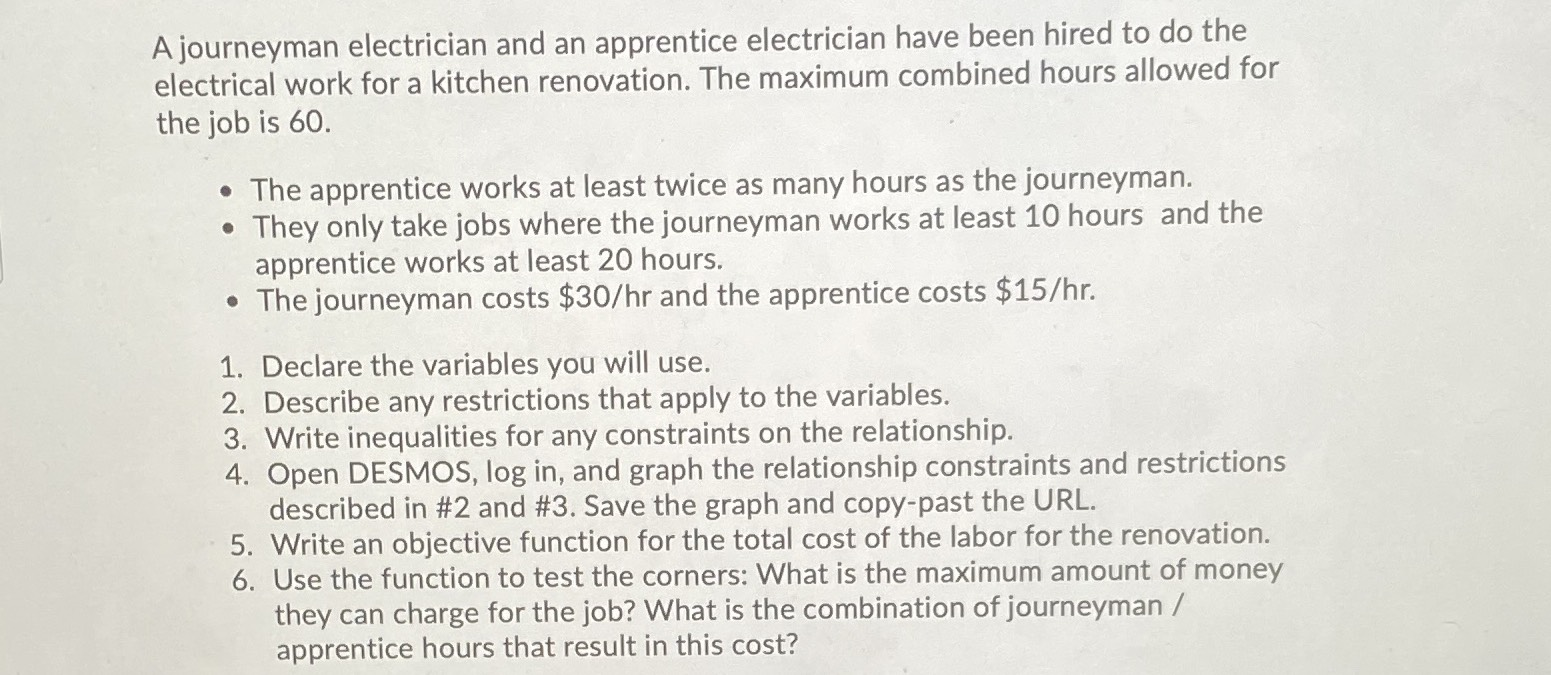### ¿Todavía tienes preguntas de matemáticas?

Pregunte a nuestros tutores expertos
Algebra
PreguntaA journeyman electrician and an apprentice electrician have been hired to do the electrical work for a kitchen renovation. The maximum combined hours allowed for the job is $$60$$ .

- The apprentice works at least twice as many hours as the journeyman.

- They only take jobs where the journeyman works at least $$10$$ hours and the apprentice works at least $$20$$ hours.

-The journeyman costs $$\ 30 / h r$$ and the apprentice costs $$\ 15 / hr$$ .

1. Declare the variables you will use.

2. Describe any restrictions that apply to the variables.

3. Write inequalities for any constraints on the relationship.

4. Open DESMOS, log in, and graph the relationship constraints and restrictions described in #2 and #3. Save the graph and copy-past the URL.

5. Write an objective function for the total cost of the labor for the renovation.

6. Use the function to test the corners: What is the maximum amount of money they can charge for the job? What is the combination of journeyman / apprentice hours that result in this cost?

$$\left\{\begin{matrix} x+ y= 60\\ y\ge 2x\\ x\ge10\\ y\ge 20 \end{matrix}\right.$$